# 高校内超市满意度调查研究Research on Supermarket Satisfaction in Universities

• 全文下载: PDF(4369KB)    PP.296-312   DOI: 10.12677/MM.2018.83035
• 下载量: 349  浏览量: 677

Supermarkets in university are special. In order to promote the development of supermarkets in campus, this article studied the satisfaction of northern area supermarket in the Ocean University of China by sending questionnaire and basing on the ACSI model theory. The evaluation system of customers’ satisfaction is divided into four main indexes: general evaluation objective “Customers” satisfaction index’ is at the first level index; six general elements in the customers’ satisfaction index model including customer expectation, perceived quality, perceived value, customer satisfaction, customer complaints and customer loyalty are at the second level; indexes which are expanded from second level indexes and are accorded with the characteristic of supermarket are at the third level; questions in the questionnaire which are expanded from third level indexes are at the fourth level. This article synthesizes analytic hierarchy process method and principal component analysis method to confirm the contribution of indexes at each level to customers’ satisfaction. In the end, this article calculated the students’ satisfaction of supermarket in the campus and put forward suggestions according to the factors that influence the satisfaction.

1. 问题的研究背景

2. 研究步骤

2.1. 建立模型

2.2. 确定指标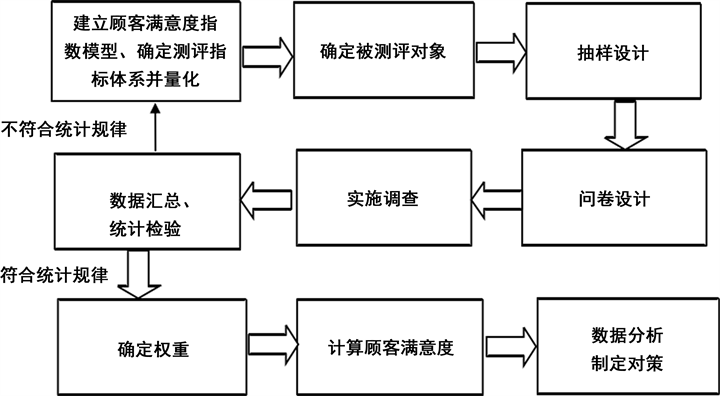Figure 1. Research process

2.3. 确定被测评对象

2.4. 问卷设计

2.5. 实施调查

2.6. 数据汇总，统计检验

2.7. 确定权重

2.8. 计算顾客满意度

$CSD=\underset{i=1}{\overset{n}{\sum }}{W}_{i}{X}_{i}\text{\hspace{0.17em}}\left(i=1,2,3,\cdots ,n\right)$ (1)

2.9. 数据分析，制定对策

3. 信度分析，效度分析

3.1. 信度分析

3.2. 效度分析

3.2.1. 内容效度Table 1. The total Cornbach α coefficient of questionnairesTable 2. The Cornbach α coefficient of secondary indicators

3.2.2. 结构效度

4. 模型的建立与求解

4.1. AHP模型构建及权重的确定

4.1.1. 建立递阶层次结构模型Table 3. The result of KMO measure and Bartlett test of sphercityTable 4. Rotated Component Matrix

4.1.2. 构造判断矩阵

$B=\left(\begin{array}{cccc}1.00& 0.75& 1.50& 1.00\\ 1.33& 1.00& 1.33& 1.33\\ 0.67& 0.50& 1.00& 0.67\\ 1.00& 0.75& 1.5& 1.00\end{array}\right)$ (2)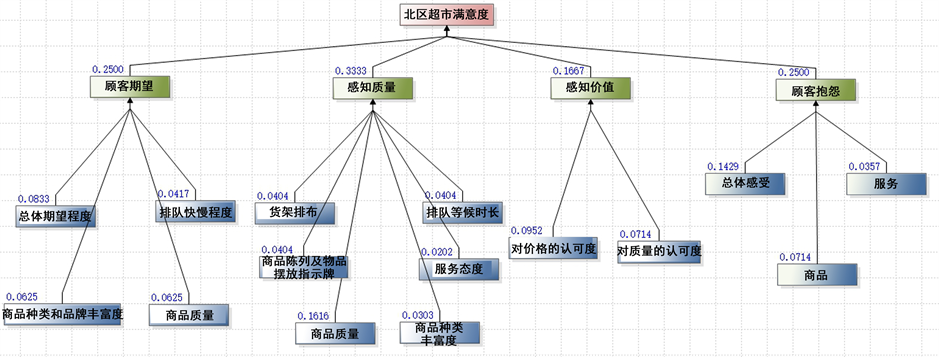Figure 2. The model of analytic hierarchy process structureFigure 3. Judgment matrix and factors’ degree of importance

${C}_{1}=\left(\begin{array}{cccc}1.00& 1.33& 2& 1.33\\ 0.75& 1.00& 1.50& 1.00\\ 0.50& 0.67& 1.00& 0.67\\ 0.75& 1.00& 1.50& 1.00\end{array}\right)$ (3)

${C}_{2}=\left(\begin{array}{cccccc}1.00& 1.00& 1.00& 2.00& 0.25& 1.33\\ 1.00& 1.00& 1.00& 2.00& 0.25& 1.33\\ 1.00& 1.00& 1.00& 2.00& 0.25& 1.33\\ 0.50& 0.50& 0.50& 1.00& 0.125& 0.67\\ 4.00& 4.00& 4.00& 8& 1.00& 5.33\\ 0.75& 0.75& 0.75& 1.50& 1.875& 1.00\end{array}\right)$ (4)

${C}_{3}=\left(\begin{array}{cc}1.00& 1.33\\ 0.75& 1.00\end{array}\right)$ (5)

${C}_{4}=\left(\begin{array}{ccc}1.00& 4.00& 2\\ 0.25& 1.00& 0.50\\ 0.50& 2.00& 1.00\end{array}\right)$ (6)

4.1.3. 一致性检验

${W}_{i}={\left(\begin{array}{cccc}0.25& 1.33& 0.167& 0.25\end{array}\right)}^{\text{T}}$ (7)

$C.R=\frac{{\lambda }_{\mathrm{max}}-n}{\left(n-1\right)R.I}$ (8)

$R.I=0.9$ (9)

$C.R=0<0.1$ (10)

4.1.4. 计算权重Table 6. The rank and weight of factors in layerTable 7. The rank and weight of factors in the first middle layerTable 8. The satisfaction of supermarket Consistency ratio: 0.0000; Weight: 1.0000; λ max :4.0000Table 9. The expectation of customers Consistency ratio: 0.0000; Weight: 0.2500; λ max :4.0000Table 10 . The perceived quality of customers Consistency ratio: 0.0000; Weight: 0.3333; λ max :6.0000Table 11 . The perceived value Consistency ratio: 0.0000; Weight: 0.1667; λ max :2.0000Table 12. The complain of customers Consistency ratio: 0.0000; Weight: 0.2500; λ max :3.0000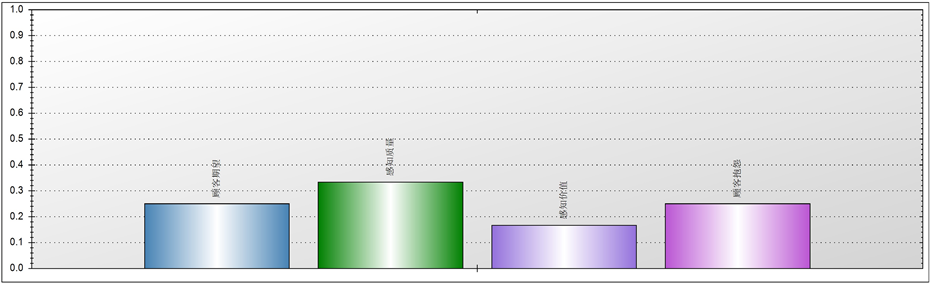Figure 4. The weight of second degree factors to satisfaction of supermarket

$\begin{array}{c}W=0.0833{x}_{1}+0.0625{x}_{2}+0.0417{x}_{3}+0.0625{x}_{4}+0.0404{x}_{5}\\ \text{\hspace{0.17em}}\text{\hspace{0.17em}}+0.0404{x}_{6}+0.0404{x}_{7}+0.0202{x}_{8}+0.1616{x}_{9}+0.0303{x}_{10}\\ \text{\hspace{0.17em}}\text{\hspace{0.17em}}+0.0952{x}_{11}+0.0714{x}_{12}+0.1429{x}_{13}+0.0714{x}_{14}+0.0357{x}_{15}\end{array}$ (11)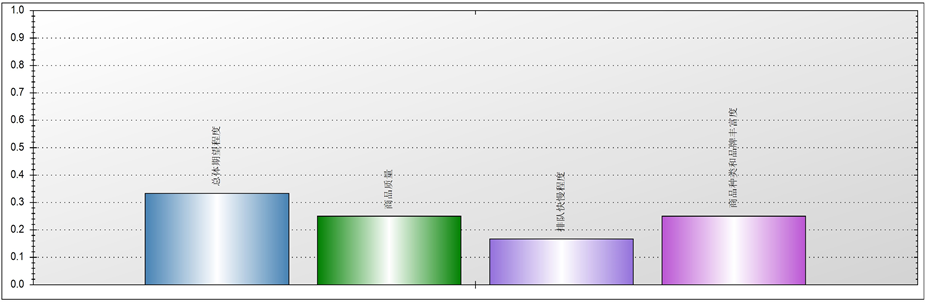Figure 5. The weight of third degree factors to the expectation of customers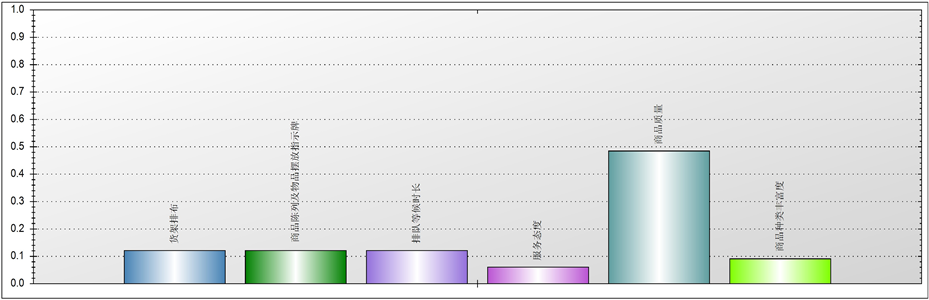Figure 6. The weight of third degree factors to the perceived qualityFigure 7. The weight of third degree factors to the perceived value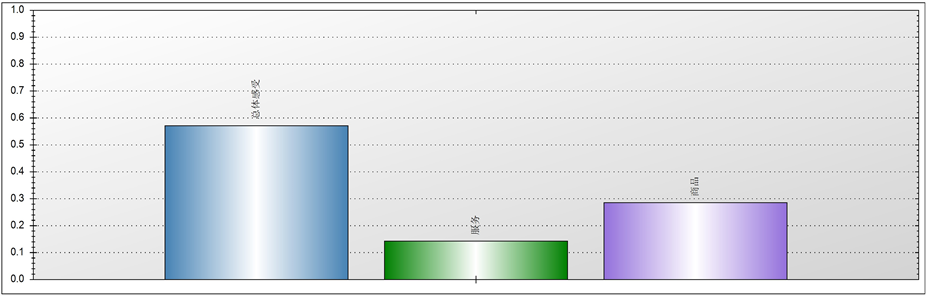Figure 8. The weight of third degree factors to the complain of customers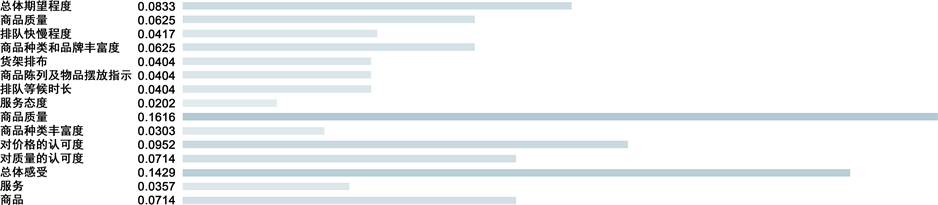Figure 9. The weight of factors

4.2. 主成分分析模型

4.2.1. 模型建立

${x}_{1},{x}_{2},\cdots ,{x}_{13}$ 分别表示13个问题对应的满意度影响因素，假设收到n份问卷。用 $i=1,2,\cdots ,n$ 分别表示第i份问卷，第i份问卷的 ${x}_{1},{x}_{2},\cdots ,{x}_{13}$ 的取值分别记作 $\left[{a}_{i1},{a}_{i2},\cdots ,{a}_{i13}\right]$ ，构造矩阵 $A={\left({a}_{ij}\right)}_{n×13}$ 。基于主成分分析的评价步骤如下。

1) 标准化处理

${\stackrel{¯}{a}}_{ij}=\frac{{a}_{ij}-{\mu }_{j}}{{s}_{ij}},\text{\hspace{0.17em}}\text{\hspace{0.17em}}i=1,2,3,\cdots ,n;j=1,2,\cdots ,14$ #(12)

${\mu }_{j}=\frac{1}{n}{\sum }_{i=1}^{n}{a}_{ij}$ (13)

${s}_{j}=\sqrt{\frac{1}{n-1}{\sum }_{i=1}^{n}{\left({a}_{ij}-{\mu }_{j}\right)}^{2}},\text{\hspace{0.17em}}\text{\hspace{0.17em}}j=1,2,\cdots ,13$ (14)

${\stackrel{¯}{x}}_{j}=\frac{{x}_{j}-{\mu }_{j}}{{s}_{j}},\text{\hspace{0.17em}}\text{\hspace{0.17em}}j=1,2,\cdots ,13$ #(15)

2) 计算相关系数矩阵R

${r}_{ij}=\frac{{\sum }_{k=1}^{n}{\stackrel{¯}{a}}_{ki}\cdot {\stackrel{¯}{a}}_{kj}}{n-1},\text{\hspace{0.17em}}\text{\hspace{0.17em}}i,j=1,2,\cdots ,13$ #(16)

3) 计算特征值与特征向量

$\begin{array}{l}{y}_{1}={u}_{11}{\stackrel{¯}{x}}_{1}+{u}_{21}{\stackrel{¯}{x}}_{2}+\cdots +{u}_{14,,1}{\stackrel{¯}{x}}_{13}\\ {y}_{2}={u}_{12}{\stackrel{¯}{x}}_{1}+{u}_{22}{\stackrel{¯}{x}}_{2}+\cdots +{u}_{14,2}{\stackrel{¯}{x}}_{13}\\ \text{\hspace{0.17em}}\text{\hspace{0.17em}}\text{\hspace{0.17em}}\text{\hspace{0.17em}}\text{\hspace{0.17em}}\text{\hspace{0.17em}}\text{\hspace{0.17em}}\text{\hspace{0.17em}}\text{\hspace{0.17em}}\text{\hspace{0.17em}}\text{\hspace{0.17em}}\text{\hspace{0.17em}}\text{\hspace{0.17em}}\text{\hspace{0.17em}}\text{\hspace{0.17em}}\text{\hspace{0.17em}}\text{\hspace{0.17em}}\text{\hspace{0.17em}}⋮\\ {y}_{14}={u}_{1,13}{\stackrel{¯}{x}}_{1}+{u}_{2,13}{\stackrel{¯}{x}}_{2}+\cdots +{u}_{13,13}{\stackrel{¯}{x}}_{13}\end{array}$ (17)

4) 选择p个主成分，计算综合评价值

${b}_{j}=\frac{{\lambda }_{j}}{{\sum }_{k=1}^{13}{\lambda }_{k}},\text{\hspace{0.17em}}\text{\hspace{0.17em}}j=1,2,\cdots ,13$ #(18)

${\alpha }_{p}=\frac{{\sum }_{k=1}^{p}{\lambda }_{k}}{{\sum }_{k=1}^{13}{\lambda }_{k}}$ #(19)

$Z=\underset{j=1}{\overset{p}{\sum }}{b}_{j}{y}_{j}$ #(20)

4.2.2. 模型求解

$Z=0.4031{y}_{1}+0.1776{y}_{2}+0.0669{y}_{3}+0.0597{y}_{4}+0.0586{y}_{5}+0.0446{y}_{6}+0.0434{y}_{7}+0.0326{y}_{8}$ (20)

5. 针对超市满足顾客需求的各方面的改进建议

5.1. 针对货架排布问题

1) 由于文具类和洗漱类商品处人流较稀疏，而食品区尤其是酸奶区人流较密集，给人以紧密感。故应适当缩小文具类售卖区域的货架间距，节约空间给予食品区，实现更好地空间分布与利用。

2) 可以适当减少同种商品的摆放数量从而使货架排列变得稀疏。对于某些饮品的摆放(例如矿泉水)，可以减少它们摆放在货架上的数量，由1排4列变为1排2列并采取多次进货的方法弥补数量上的减少。也可适当撤除一些货架，用增加补货次数和频率来实现商品的正常供应。

3) 可以选择在人流量较小的时候(如下午2:00~3:00，早晨9:00~11:00)进货，避开高峰期的同时又可以使商品数量得到满足，同时还可以使货架排列更稀疏。

4) 另外工作人员在超市内宣传新商品时也会占据一定的空间，为了避免拥堵，可以不用工作人员在超市内宣传商品，而改用超市的广播宣传新的商品。目前超市中放的音乐声音较小，特别是在高峰期时，超市内环境嘈杂，放音乐的效果并不明显，故可以将音乐改为播放宣传语以达到腾出空间，宣传商品的目的。Table 13. The results of principal component analysis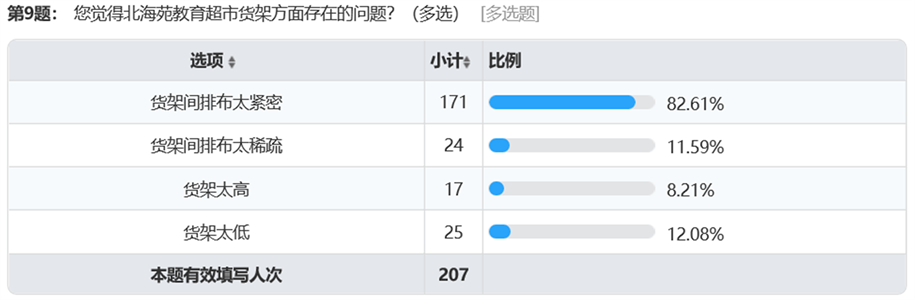Figure 10. The findings of shelf arrangement

5.2. 针对商品排布问题

1) 更改指示牌排放位置，由目前的挂在天花板上改为立在货架上方。对于目前的方式，有些指示牌放置太高，离货架比较远，所以看起来不明显。所以需要降低高度，将指示牌放置在较低的位置(货架上方)。对于有些较为明显的产品(如牛奶、酸奶、饮料类等)也可以选择不设置指示牌以便节约空间，但是对于一些冷门商品(如特殊零食类)需要设立较为明显的指示牌。也可以选择增加地贴，指示同学们寻找商品时的方向。

2) 尽量将商品分类合理，将热门商品设置处分散开，避免过于集中造成拥挤。

5.3. 针对商品丰富度问题

5.4. 对于网红商品和现场加工零食的引入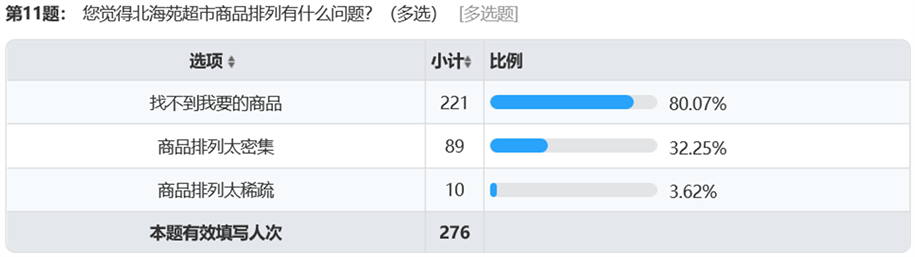Figure 11. The findings of commodity arrangement (1)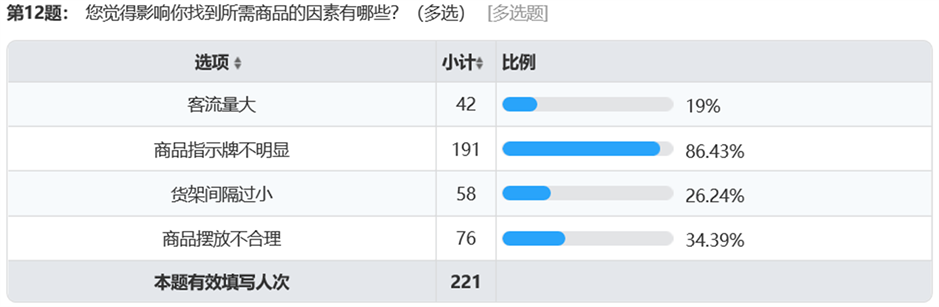Figure 12. The findings of commodity arrangement (2)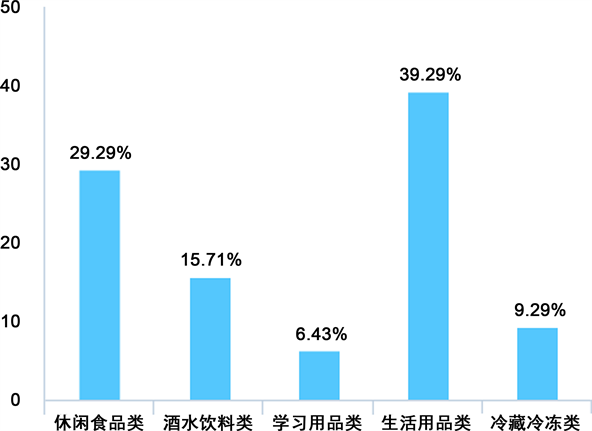Figure 13. The findings of commodity abundance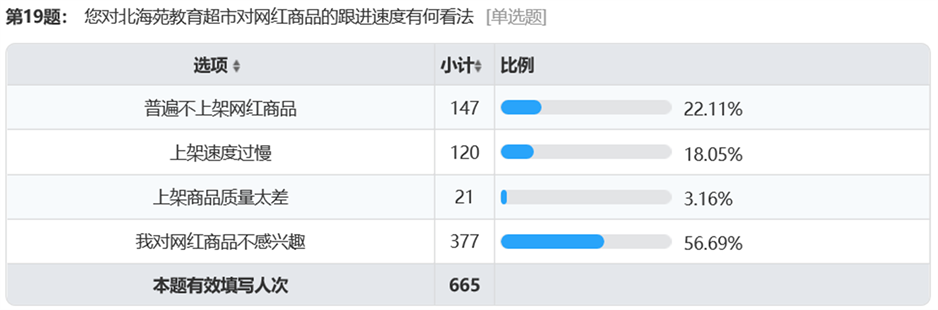Figure 14. The findings of red net goods

5.5. 对特定小型商品引进新的结账方式

5.6. 对会员卡引入方面

  沈涵. 基于ACSI的经济型酒店顾客满意度测评模型[J]. 旅游学刊, 2011, 26(1): 58-62.  张辉辉. 基于ACSI模型的客户满意度测评体系研究[D]: [硕士学位论文]. 长春: 吉林财经大学, 2016.  马文军, 潘波. 问卷的信度和效度以及如何用SAS软件分析[J]. 中国卫生统计, 2000, 17(6): 364-365.  苏中华, 李四劝, 成义仁. 量表评估的内部一致性与克朗巴赫α系数的应用评价[J]. 临床心身疾病杂志, 2009, 15(1): 85-86.  司守奎, 孙兆亮. 数学建模算法与应用[M]. 第2版. 北京: 国防工业出版社, 2015.